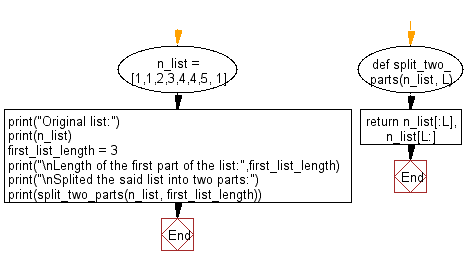﻿ Python: Split a given list into two parts where the length of the first part of the list is given - w3resource# Python: Split a given list into two parts where the length of the first part of the list is given

## Python List: Exercise - 78 with Solution

Write a Python program to split a given list into two parts where the length of the first part of the list is given.

Sample Solution:

Python Code:

``````def split_two_parts(n_list, L):
return n_list[:L], n_list[L:]
n_list = [1,1,2,3,4,4,5, 1]
print("Original list:")
print(n_list)
first_list_length = 3
print("\nLength of the first part of the list:",first_list_length)
print("\nSplited the said list into two parts:")
print(split_two_parts(n_list, first_list_length))

```
```

Sample Output:

```Original list:
[1, 1, 2, 3, 4, 4, 5, 1]

Length of the first part of the list: 3

Splited the said list into two parts:
([1, 1, 2], [3, 4, 4, 5, 1])
```

Flowchart:## Visualize Python code execution:

The following tool visualize what the computer is doing step-by-step as it executes the said program:

Python Code Editor:

Have another way to solve this solution? Contribute your code (and comments) through Disqus.

What is the difficulty level of this exercise?

Test your Python skills with w3resource's quiz

﻿

## Python: Tips of the Day

Floor Division:

When we speak of division we normally mean (/) float division operator, this will give a precise result in float format with decimals.

For a rounded integer result there is (//) floor division operator in Python. Floor division will only give integer results that are round numbers.

```print(1000 // 300)
print(1000 / 300)```

Output:

```3
3.3333333333333335```